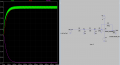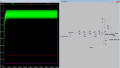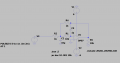# Choice of resistor value for unit gain amp affecting output

#### samihawasli

Joined Oct 19, 2020
10
Hello,

I am trying to create a steady DC output to drive some pHEMTS, HMC7357. To control the gates I need to produce -1.4, and -0.8V and supply about 0.5-1 mA. To do so, I was planning on using a filtered PWM output from a microcontroller, followed by a -1 inverting op-amp circuit. I was playing around in LT-spice and i got some results I didn't expect. Perhaps someone can help me understand what is happening and why.Above is a screen capture of my schematic and simple transient analysis. I am using a op-amp model from the TI website for the LM358. The input is a 500 Hz 50% duty cycle square wave with a magnitude of 5V. I am not plotting the input on the graph because the X axis time scale is long. The circuit acts as one expects, the PWM signal is filtered at each stage, V1,V2 and the output, Vout, is a steady -2.5V.However, when i change the value of the feedback resistors, now 1K instead of 1 Meg, the value of the output changes drastically. From what I understand about IDEAL op-amps it shouldn't really matter what value of feedback resistors I use? clearly I am missing something fundamental to op-amps.

BONUS question: I tested the first circuit, with 1Meg feedback, on a breadboard. Without the pHEMT connected, I got the -1.4 and -0.8V as simulated and expected. Connecting the circuit to the pHEMT's gate:
With no driving power to the pHEMT, meaning virtually no current DC to the gate, the outputs work as expected.
When I apply a RF power, ~20 dBm causing at most 1mA gate current, the PWM output from the AVG drops, subsequently dropping both of my voltages, expected -0.8V to -0.7V and -1.4 to -1.3! None of my circuit elements should be drawing much, if ANY current, does anyone have any clues?

Happy to provide and more context,
Thanks for any and all help,
Sami

#### AlbertHall

Joined Jun 4, 2014
12,020
Because of the negative feedback, the inverting input of the amplifier is a virtual groud - at 0V.

In the first circuit you have 2x 10k resistors and then a 1M resistor connected to this 0V point.
In the second circuit the 2 x 10k resistors have just 1k connected to 0V. This severely loads down the signal from the input resistors.

#### samihawasli

Joined Oct 19, 2020
10
Because of the negative feedback, the inverting input of the amplifier is a virtual groud - at 0V.

In the first circuit you have 2x 10k resistors and then a 1M resistor connected to this 0V point.
In the second circuit the 2 x 10k resistors have just 1k connected to 0V. This severely loads down the signal from the input resistors.
Was my understanding that the inputs to op-amps draw virtually no current? With no current no voltage drop?

#### AlbertHall

Joined Jun 4, 2014
12,020
The amplifier inputs draw near zero current but are we agreed that the inverting amplifier input is at zero volts?
See https://www.electronics-tutorials.ws/opamp/opamp_2.htmlAnd that current i must flow through the 10k input resistors and will drop voltage across those resistors.
Or to put it another way, Rin in the above diagram consists of 2x 10K + 1k = 21k. The feedback resistor is 1k. There fore the gain is -1/21.

#### Audioguru again

Joined Oct 21, 2019
4,673
The input two 10k resistors feeding your 1k opamp input resistor attenuate the input level to 1/21 times which is 4.76%.
The inputs of opamps draw virtually no current, but the 1k resistor does.

#### Ian0

Joined Aug 7, 2020
5,086
The input doesn't draw any (significant amount of) current.
If V2 is at 1.4V and the output is at -1.4V, then a current will flow between the two points, equal to 2.8V divided by 2k.
The inverting input is therefore halfway between 1.4V and -1.4V, which is 0V. It's at 0V even though it's not connected to 0V. That's why it's called a "virtual earth".

#### Ian0

Joined Aug 7, 2020
5,086
Hello,

I am trying to create a steady DC output to drive some pHEMTS, HMC7357. To control the gates I need to produce -1.4, and -0.8V and supply about 0.5-1 mA. To do so, I was planning on using a filtered PWM output from a microcontroller, followed by a -1 inverting op-amp circuit. I was playing around in LT-spice and i got some results I didn't expect. Perhaps someone can help me understand what is happening and why.
I'd suggest you use a MFB filter.
GO here: http://sim.okawa-denshi.jp/en/OPtazyuLowkeisan.htm and scroll down to Calculate the R and C values for the multiple feedback filter at a given frequency and Q factor. Type in about 50Hz in and leave the Q at 0.7.

•samihawasli

#### samihawasli

Joined Oct 19, 2020
10
I'd suggest you use a MFB filter.
GO here: http://sim.okawa-denshi.jp/en/OPtazyuLowkeisan.htm and scroll down to Calculate the R and C values for the multiple feedback filter at a given frequency and Q factor. Type in about 50Hz in and leave the Q at 0.7.
Thanks, that is a good solution to my problem. One more follow up question if you don't mind.Using components I had available, I built and simulated the circuit above. It worked as expected, but when I connected it to the gates of the amplifier, something rather strange happened. For reference, Vdrain for the amplifier is 6V.
1) Controlling the amp's gate using a benchtop power supply, applying a gate voltage Vg = -1.4 yields a drain current Id = 13mA. Vg = -1.1 yields Id = 239mA, and Vg = -0.8 yields Id = 513mA
2) Controlling the amp's gates using the above circuit and PWM inputs; output voltages similar to voltage levels in step 1 yields Id = 20mA, 427mA, and 1093mA, almost twice the current at each current level!
I hope that makes sense, any clue as to why this might be happening?